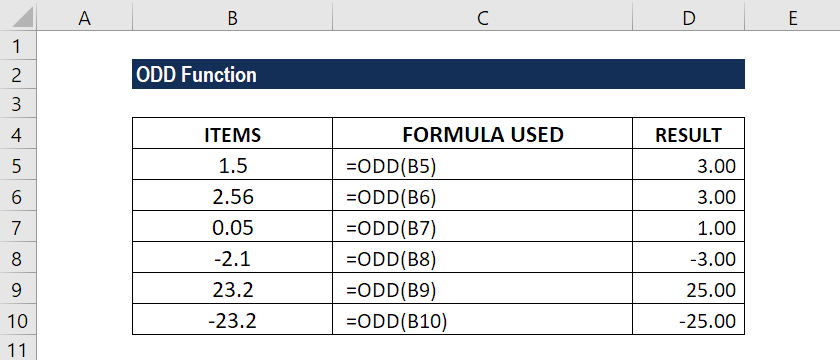# ODD Function

Rounds a specified number away from zero to the next odd number

## What is the ODD Function?

The ODD function is classified as an Excel Math and Trigonometry function. It will round a specified number away from zero to the next odd number. The function will round a positive number up and a negative number down.

As a financial analyst, ODD is a very useful function, as it helps round a number and makes it an odd number. It eliminates the least significant digits, simplifying the notation but keeping close to the original value. The function was introduced in MS Excel 2000.

### Formula

=ODD(number)

The ODD function uses the following argument:

1. Number (required argument) – This is the value that we wish to round to the nearest odd number.

### How to use the ODD Function in Excel?

To understand the uses of the ODD function, let’s consider an example:

#### Example 1

Suppose we are given the following data:

ITEMSFORMULA USEDREMARKS
1.5 =ODD(B5)3
2.56 =ODD(B6)3
0.05 =ODD(B7)1
-2.1 =ODD(B8)-3
23.2 =ODD(B9)25
-23.2 =ODD(B10)-25

We get the results below:### Things to remember about the ODD Function

1. #VALUE! error – Occurs when the supplied number argument is not recognized as a numeric value by MS Excel.
2. As the function always rounds numbers up, which is away from zero, a positive number will become larger and negative number will become a smaller number that is more negative.
3. The function will always skip even integers.

Thanks for reading CFI’s guide to important Excel functions! By taking the time to learn and master these Excel functions, you’ll significantly speed up your financial analysis. To learn more, check out these additional CFI resources:

• Excel Functions for Finance